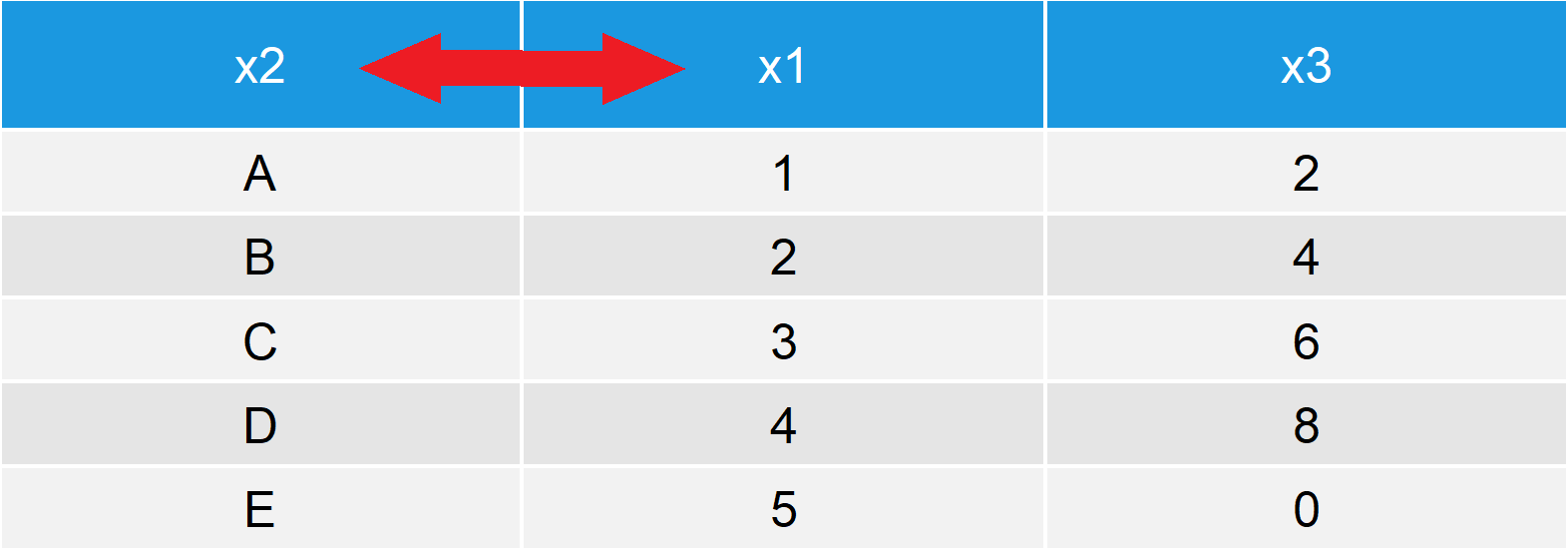# Reorder Columns of Data Frame in R (4 Examples)

In this tutorial, I’ll explain how to manually order the variables of a data frame in the R programming language.

The tutorial will contain these topics:

Let’s do this.

## Creation of Example Data

In this tutorial, I’ll use the following example data frame:

```data <- data.frame(x1 = 1:5,              # Create example data
x2 = LETTERS[1:5],
x3 = c(2, 4, 6, 8, 0))
data                                      # Print example data```Table 1: Example Data Frame in R.

Table 1 illustrates the structure of our data: It contains five rows and the three columns/variables x1, x2, and x3.

Let’s reorder these variables…

## Example 1: Reorder Columns of Data Frame by Index

In the first example, you’ll learn how to reorder data frame columns by their index (i.e. the position of the variable within the data frame).

We simply have to open a squared bracket (i.e. []), write a comma (i.e. ,) to tell R that we want to change the columns, and specify a vector with the new ordering that we want to enforce (i.e. c(2, 1, 3)):

`data[ , c(2, 1, 3)]                       # Reorder columns by index`Table 2: Reordered Data Frame.

Table 2 shows the reordered data frame. We moved the variable x2 to the first position and the variable x1 to the second position.

That’s in my opinion the easiest way how to reorder data frames in R. However, there are several other options that I want to show you in the remaining tutorial. So keep on reading…

## Example 2: Reorder Columns of Data Frame by Variable Name

The second example is similar to Example 1, but this time we are ordering our data frame columns by their name:

`data[ , c("x2", "x1", "x3")]              # Reorder columns by names`

Have a look at your RStudio console. The previous code is producing exactly the same output as Example 1.

## Example 3: Reorder Columns of Data Frame with subset Function

Another alternative for changing the order of variables is provided by the subset function of the base installation of R. We can apply the subset function as follows:

`subset(data, select = c(2, 1, 3))         # Reorder columns with subset()`

Again, the same output.

## Example 4: Reorder Columns of Data Frame with select Function of dplyr Package

Many R programmers prefer to manipulate their data based on the dplyr package of the tidyverse environment. Let’s install and load dplyr to R:

```install.packages("dplyr")                 # Install dplyr package

Now, we can use the select function of the dplyr package to sort our data frame columns as follows:

`data %>% select(x2, x1, x3)               # Reorder columns with select()`

The output is the same as in the previous examples.

## Video, Further Resources & Summary

Have a look at the following video on my YouTube channel. I’m explaining how to swap variables around in a data frame based on the R codes of this article in the video.

Furthermore, I can recommend having a look at the related tutorials of www.statisticsglobe.com. You can find some tutorials about the manipulation of data frames and matrices below:

In summary: On this page, I explained how to shift data frame columns to a different position in small or large data frames in R programming. In case you have any additional questions, let me know in the comments below.

Subscribe to the Statistics Globe Newsletter